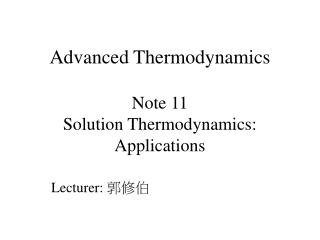Download PresentationAdvanced Thermodynamics Note 11 Solution Thermodynamics: Applications# Advanced Thermodynamics Note 11 Solution Thermodynamics: Applications - PowerPoint PPT Presentation

Download Presentation##### Advanced Thermodynamics Note 11 Solution Thermodynamics: Applications

Download Policy: Content on the Website is provided to you AS IS for your information and personal use and may not be sold / licensed / shared on other websites without getting consent from its author. While downloading, if for some reason you are not able to download a presentation, the publisher may have deleted the file from their server.

- - - - - - - - - - - - - - - - - - - - - - - - - - - E N D - - - - - - - - - - - - - - - - - - - - - - - - - - -
##### Presentation Transcript

1. Advanced ThermodynamicsNote 11Solution Thermodynamics: Applications Lecturer: 郭修伯

2. Liquid-phase properties from VLE data • Fugacity • For species i in the vapor mixture: • Vapor/liquid equilibrium: • The vapor phase is assumed an ideal gas: • Therefore: • The fugacity of species i (in both the liquid and vapor phases) is equal to the partial pressure of species i in the vapor phase. • Its value increases from zero to Pisat for pure species i

3. Fig. 12.1 Table 12.1 The first three columns are P-x1-y1 data. Columns 4 and 5 are: Column 6 is:

4. Fig 12.3 Fig 12.2 Henry’s constant, the limiting slope of the curve at xi = 0. Henry’s law expresses: , it is approximate valid for small values of xi

5. Henry’s law Lewis/Randall rule Gibbs/Duhem equation x2→ 1 x1→ 0 Gibbs/Duhem equation for binary mixture at const. T and P: The Lewis/Randall rule, Division by dx1 when x1 = 1, limit

6. Excess Gibbs energy • Table 12.2 • Column 6:

7. Fig. 12.5 Positive deviation from Raoult’s law behavior: The dimensionless excess Gibbs energy: The value of GE/RT is zero at both x1= 0 and x1 =1

8. From Fig 12.5(b), linear relation: Similarly, The Margules equations Limiting conditions:

9. VLE data for diethyl ketone (1) / n-hexane (2) at 65°C are given in the first three columns of Table 12.4. Reduce the data. Table 12.4 Fig 12.7 The solid lines. Not consistency! Omit Barker’s method Fig 12.7(b) for (GE/x1x2RT) fitting:

10. Models for the excess Gibbs energy weak • GE/RT = f (T, P, composition) • At constant T: Data fitting, convenient, but only for binary system The Redlich/Kister expansion The Margules equation The van Laar equation

11. Local composition models • Can be applied to multi-component systems • The Wilson equation: • The NRTL(Non-Random-Two-Liquid) equation: • The UNIQUAC equation and the UNIFAC method: • App. H.

12. Property changes of mixing • Excess properties The M change of mixing Because of their direct measurability, V and H are the property changes of mixing of major interest.

13. Fig 12.10

14. The excess enthalpy (heat of mixing) for liquid mixture of species 1 and 2 at fixed T and P is represented by the equation: Determine expressions for and as functions of xi. The partial properties:

15. Fig 12.13 1. Each ΔM is zero for a pure species. 2. The Gibbs energy change of mixing ΔG is always positive. 3. The entropy change of mixing ΔS is positive.

16. Heat effects of mixing processes • Heat of mixing: • For binary systems: • When a mixture is formed, a similar energy change occurs because interactions between the force fields of like and unlike molecules are different. • Heat of solution • based on 1 mol of solute dissolve in liquids:

17. Calculate the heat of formation of LiCl in 12 mol of H2O at 25°C. Fig 12.14

18. A single-effect evaporator operating at atmospheric pressure concentrates a 15% (by weight) LiCl solution to 40%. The feed enters the evaporator at the rate of 2 kg/s at 25°C. The normal boiling point of a 40% LiCl solution is about 132°C, and its specific heat is estimated as 2.72 kJ/kg °C. What is the heat transfer rate in the evaporator? Feed at 25°C 2 kg/s 15% LiCl 1.25 kg superheated steam at 132°C and 1 atm 0.75 kg 40% LiCl at 132°C Q The energy balance: the total enthalpy of the product streams minus the total enthalpy of the feed stream liquid water is vaporized and heated to 132°C 0.75 kg of 40% LiCl solution is heated to 132°C mixing of 0.45 kg of water with 0.30 kg of LiCl(s) to form a 40% solution at 25°C separation of 2 kg of a 15% LiCl solution into its pure constituents at 25°C

19. Enthalpy/concentration diagrams • The enthalpy/concentration (Hx) diagram Fig 12.17

20. Solid NaOH at 70°F is mixed with H2O at 70°F to produce a solution containing 45% NaOH at 70°F. How much heat must be transferred per pound mass of solution formed? Energy balance: 45% NaOH: on the basis of 1 (lbm): 0.45(lbm) of solid NaOH dissolved in 0.55 (lbm) of H2O. Fig 12.19, x1 = 0: Fig 12.19, x1 = 45%: